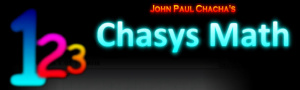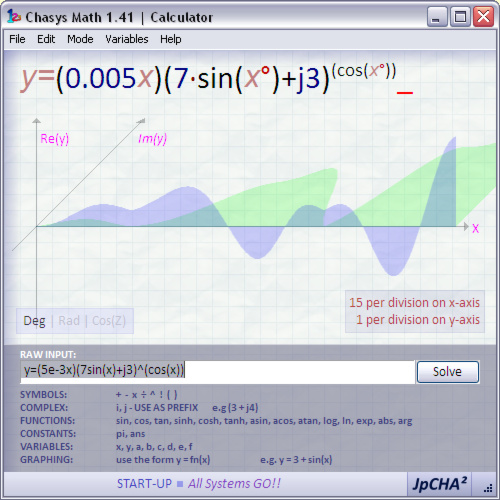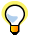...

### Chasys Math Help:Introduction to CalculatorWhat is Chasys Math Calculator? Chasys Math Calculator is the expression evaluation and function plotting component of Chasys Math. It allows you to solve long, complicated equations, and does so for both real and complex numbers. These can also be plotted o a graph. The application provides the user with user variables and all functions supported by modern scientific calculators.Input is Super Visually Perfect Algebraic Method, which makes input very easy to use and understand. Besides, the application displays it's interpretation of the user's input, so that ambiguities and errors can be recognized immediately.The help file for this application is still a bit sketchy, as this is a very new app. Kindly bear with me. Thank You!Created by John Paul Chacha's Lab, 2008